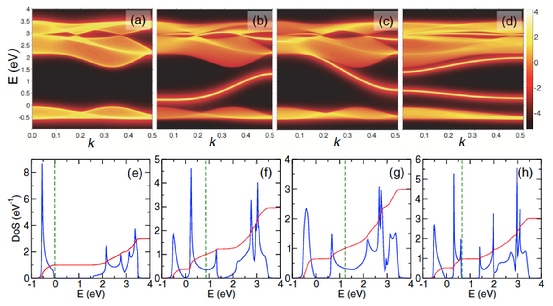# 31-05-2016 Publication in PRB by Mojtaba Farmanbar, Taher Amlaki, and Geert Brocks

Green’s function approach to edge states in transition metal dichalcogenides

Mojtaba Farmanbar, Taher Amlaki, and Geert Brocks Physical Review B93, 205444 (2016).

The semiconducting two-dimensional transition metal dichalcogenides MX2 show an abundance of one-dimensional metallic edges and grain boundaries. Standard techniques for calculating edge states typically model nanoribbons, and require the use of supercells. In this paper, we formulate a Green’s function technique for calculating edge states of (semi-)infinite two-dimensional systems with a single well-defined edge or grain boundary. We express Green’s functions in terms of Bloch matrices, constructed from the solutions of a quadratic eigenvalue equation. The technique can be applied to any localized basis representation of the Hamiltonian. Here, we use it to calculate edge states of MX2 monolayers by means of tight-binding models. Aside from the basic zigzag and armchair edges, we study edges with a more general orientation, structurally modifed edges, and grain boundaries. A simple three-band model captures an important part of the edge electronic structures. An 11-band model comprising all valence orbitals of the M and X atoms is required to obtain all edge states with energies in the MX2 band gap. Here, states of odd symmetry with respect to a mirror plane through the layer of M atoms have a dangling-bond character, and tend to pin the Fermi level.Figure 1 (a) k-resolved density of states (DOS) per MoS2 unit of bulk MoS2 in the three-band tight-binding model, with the k vector parallel to the zigzag edge, and the zero of energy at the top of the valence band. The DOS is plotted on a logarithmic scale (right-hand side n denotes amplitude 10−n) using a broadening parameter η = 0.05 eV. (b), (c), (d) k-resolved DOSs of the Mo edge, the S edge, and the armchair edge, respectively. (e), (f), (g), (h) k-integrated DOS per MoS2 unit of bulk MoS2, the Mo edge, the S edge, and the armchair edge, all plotted on a linear scale (η = 0.05 eV). The red solid lines give the counting function and the green dashed lines indicate the charge-neutrality level (CNL).

You can find the full publication here ...